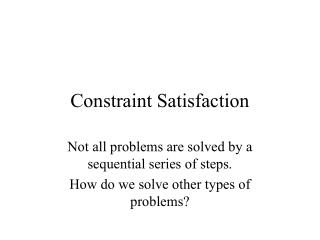DownloadDownload PresentationConstraint Satisfaction

# Constraint Satisfaction

Download Presentation## Constraint Satisfaction

- - - - - - - - - - - - - - - - - - - - - - - - - - - E N D - - - - - - - - - - - - - - - - - - - - - - - - - - -
##### Presentation Transcript

1. Constraint Satisfaction Not all problems are solved by a sequential series of steps. How do we solve other types of problems?

2. Problem structure • Some problems are structured such that you need to make a proper sequence of moves to solve the problem • Driving a car • Missionaries and cannibals • Not all are, though. For some, what matters is the end goal, not how you get there • 8 queens • Choosing a college

3. Constraint satisfaction problems • In CSPs, we are simply looking for a solution to the problem where a set of variables all have values that are valid within the set of constraints that defines the goal state. • Examples: • Map coloring • Cryptarithmetic

4. Searching in CSPs • Because the order of moves doesn’t matter, “searching” in a CSP is very different. • Instead of searching a tree that is essentially pre-defined, with each level representing a move, now we search a tree we generate, where each level contains the possible values for the variables.

5. How best to search? • One way of searching a CSP is to use our previous search algorithms. • The first step is to pick a value for one of the variables. • The next step picks a value for another variable, etc. • Problem: For the first level, the number of child nodes is the number of variables  the average number of values per variable. • This creates a tree with a huge number of nodes! Way more nodes than all the possible combinations.

6. Efficient searching in CSPs • Clearly, we only gain a benefit over testing all possible variable-value combinations if we check fewer nodes than that. • The easiest way is to have each level in the search tree correspond to assigning a value to only one variable. • But how do we decide which variable to use first? • This is an important question because if we choose poorly, we may over constrain ourselves • The goal should be to find a choice that both minimizes branchiness, while leaving us with the most options (depth).

7. Minimum Remaining Values • Choose the remaining variable with the least number of possible values. • Minimizes branchiness because this variable will have the fewest branches at a given depth of the tree. • Unclear how it affects depth since it doesn’t take into account how this choice affects other variables.

8. Degree heuristic • Choose the one that is involved in the largest number of other constraints. • Reduces branchiness by pruning the number of possibilities for other variables • Can over-prune, so works best when used primarily to break ties in the MRV heuristic

9. Least-constraining value • Once we’ve chosen a variable, how do we choose a value? • Choose the value that rules out the fewest choices (prunes the fewest branches from the tree).

10. Forward checking • So far, we’ve only looked at constraints at the time we chose a particular variable-value combination. • Wouldn’t it be easier to look ahead and see the results of given choices on the constraints? • Forward checking works by, every time we choose a value for a variable, it eliminates the values for other variables that conflict with that constraint. • In combination with MRV, yields the most efficient constraint satisfaction system.